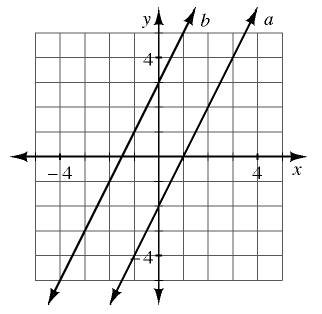### Home > INT1 > Chapter Ch2 > Lesson 2.1.3 > Problem2-30

2-30.When Yoshi graphed the lines $y=2x+3$ and $y=2x−2$, she got the graph shown at right. 2-30 HW eTool (Desmos) Homework Help ✎.

1. One of the lines at right matches the equation $y=2x+3$, and the other matches $y=2x−2$. Which line matches which equation?

In the equation y = mx + b, b is the y-intercept.
Match the b-value in each equation to the y-intercept on the graph.

2. Yoshi wants to add the line $y=2x+1$ to her graph. Predict where it would lie and sketch a graph to show its position. Justify your prediction.

The line has the same slope so it will be parallel to the other two lines. Again, use to y-intercept to decide where the line will be on the graph.

It would lie between the lines a and b because it has y = 1 as the intercept.

3. Where would the line $y=−2x+1$ lie? Again, justify your prediction and add the graph of this line to your graph from part (b).

The negative slope tells you that the line will travel downward. Once again, use the y-intercept to decide where to put the line.

Use the graph in the eTool below to solve the problem.
Click the link at the right to view full version of the eTool: Int1 2-30 HW eTool.